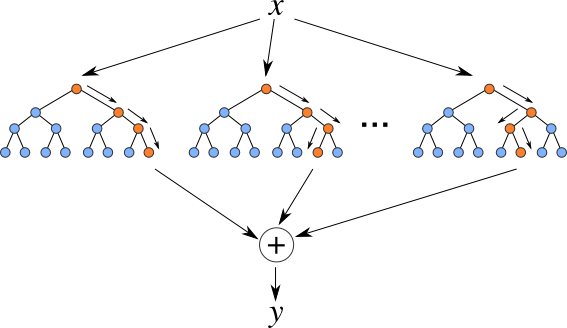Before going through this tutorial take a look at the overview section.Random forests are an ensemble learning method for classification, regression and other tasks that operate by constructing a multitude of decision trees at training time and outputting the class that is the mode of the classes (classification) or mean prediction (regression) of the individual trees. Random decision forests correct for decision trees’ habit of overfitting to their training set.

The training algorithm for random forests applies the general technique of bootstrap aggregating, or bagging, to tree learners. Given a training set `X` with responses `Y`, bagging repeatedly selects a random sample with replacement of the training set. After training, predictions for unseen samples can be made by averaging the predictions from all the individual regression trees or by taking the majority vote in the case of decision trees. Random forests can also do another bagging – feature bagging, which is a random subset of the features. The reason for doing this is the correlation of the trees in an ordinary bootstrap sample: if one or a few features are very strong predictors for the response variable (target output), these features will be selected in many of the B trees, causing them to become correlated.

## DEFINITION

• `N` is the number of data points
• `M` is the number of selected features
• `K` is the number of trees

## METHOD

The following is the procedure of Harp Random Forests training:

1. Randomly select `N` samples with replacement for `K` trees (totally `K` times).

2. Select `M` features randomly which will be used in decision tree.

3. Build decision tree based on these `M` features

4. Repeat Step 2 and 3 `K` times.

The predicting part will use these decision trees to predict `K` results. For regression, the result is the average of each tree’s result and for classification, the majority vote will be the output.

This tutorial extends a random forest implementation of a popular java machine learning library javaml from a shared memory version into a distributed version.

## Step 0 — Data Bootstraping

```// create bootstrap samples for each tree
Dataset baggingDataset = new DefaultDataset();
Random rand = new Random();
for (int i = 0; i < dataset.size(); i++) {
baggingDataset.add(dataset
.get(rand.nextInt(dataset.size())));
}
```

## Step 1 —Train forests

```// each thread build one decision tree
Classifier rf = new RandomForest(1, false,
numFeatures, new Random());
rf.buildClassifier(dataset);
```
```//private DynamicScheduler<Dataset, Classifier, RFTask> rfScheduler;

rfScheduler.start();
for (int i = 0; i < numTrees
/ numMapTasks; i++) {
Dataset baggingDataset =
Util.doBagging(trainDataset);
rfScheduler.submit(trainDataset);
}

while (rfScheduler.hasOutput()) {
rfClassifier
.add(rfScheduler.waitForOutput());

}
rfScheduler.stop();
```

## Step 2 — Synchronize majority vote

```for (Instance testData : testDataset) {
IntArray votes = IntArray.create(2, false);
for (Classifier rf : rfClassifier) {
Object classValue = rf.classify(testData);
if (classValue.toString().equals("0")) {
votes.get() += 1;
} else {
votes.get() += 1;
}
}
Partition<IntArray> partition =
new Partition<IntArray>(partitionId,
votes);
predictTable.addPartition(partition);
partitionId += 1;

}

reduce("main", "reduce", predictTable, 0);
if (this.isMaster()) {
printResults(predictTable, testDataset);

}
```

# Run example

### Data

The dataset used is sampled from Aireline dataset. Refer to the dataset directory for more details.

The format for the data should be a list of <DAY_OF_WEEK DEP_DELAY DEP_DELAY_NEW DEP_DELAY_GROUP ARR_DELAY ARR_DELAY_NEW UNIQUE_CARRIER_ID ORIGIN_STATE_ABR_ID DEST_STATE_ABR_ID CLASS>. For example,

```1 14 14 0 10 10 15 10 20 0
1 -3 0 -1 -24 0 15 10 20 0
```

### Put data on hdfs

```hdfs dfs -mkdir -p /data/airline
rm -rf data
mkdir -p data
cd data
split -l 74850 \$HARP_ROOT_DIR/datasets/tutorial/airline/train.csv
cd ..
hdfs dfs -put data /data/airline
hdfs dfs -put \$HARP_ROOT_DIR/datasets/tutorial/airline/test.csv /data/airline/
```

### Compile

Select the profile related to your hadoop version. For ex: hadoop-2.6.0. Supported hadoop versions are 2.6.0, 2.7.5 and 2.9.0

```cd \$HARP_ROOT_DIR
mvn clean package -Phadoop-2.6.0
```
```cd \$HARP_ROOT_DIR/contrib/target
cp contrib-0.1.0.jar \$HADOOP_HOME
cp \$HARP_ROOT_DIR/third_party/javaml-0.1.7.jar \$HADOOP_HOME/share/hadoop/mapreduce
cp \$HARP_ROOT_DIR/third_party/ajt-2.11.jar \$HADOOP_HOME/share/hadoop/mapreduce
cd \$HADOOP_HOME
```

### Run

```hadoop jar contrib-0.1.0.jar edu.iu.rf.RFMapCollective
Usage: edu.iu.rf.RFMapCollective <numTrees> <numMapTasks> <numThreads> <trainPath> <testPath> <outputPath>
```

### Example

```hadoop jar contrib-0.1.0.jar edu.iu.rf.RFMapCollective 32 2 16 /data/airline/data/ /data/airline/test.csv /out
```

This will start 2 mappers with 16 threads in each mapper to build 32 decision trees. The accuracy of prediction on the test dataset is the output.